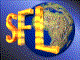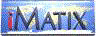| iMatix home page | << | < | > | >>SFLVersion 2.11

### compress_nulls

```#include "sflcomp.h"
word
compress_nulls (
byte *src,
byte *dst,
word src_size)
```

#### Synopsis

Similar to compress rle(), but optimised towards compression of binary zeroes. Use this when you are certain that the sparse areas are set to binary zeroes. You must use expand nulls () to decompress a block compressed with this function. Returns the size of the compressed data. The dst buffer should be 10% larger than the src buffer. The src buffer must be at least src_size + 1 bytes long. It may be modified. The compressed data contains these strings:
 [01-7F][data...] String of uncompressed data, 1 to 127 bytes. [82-FF] Run of 2 to 127 binary zeroes. [80-FF] Run of 128 to 255 binary zeroes. [lo][hi] Run of 256 to 2^16 binary zeroes. [len][byte] Run of 4 to 255 identical bytes. [lo][hi][byte] Run of 256 to 2^16 identical bytes.

#### Source Code - (sflcomp.c)

```{
word
dst_size,                       /*  Size of compressed data          */
src_scan,                       /*  Scan through source data         */
run_end,                        /*  Points to end of run of bytes    */
length = 0;                     /*  Size of the run or string        */
byte
cur_byte,                       /*  Next byte to process             */
Bool
have_run;                       /*  TRUE when we have a run          */

src_scan = 0;                       /*  Start at beginning of source     */
dst_size = 0;                       /*  No output yet                    */
header   = NULL;                    /*  No open unpacked string          */
while (src_scan < src_size)
{
cur_byte = src [src_scan++];
have_run = FALSE;               /*  Unless we find a run             */

/*  Two identical bytes may signal the start of a run                */
if (cur_byte == src [src_scan]
&&  src_scan < src_size)
{
/*  Stick-in a sentinel character to ensure that the run ends    */
src [src_size] = !cur_byte;
run_end = src_scan;         /*  src_scan <= src_size             */
while (src [run_end] == cur_byte)
run_end++;

/*  A run is 4+ identical bytes or 2+ nulls                      */
if ((run_end - src_scan > 2) || cur_byte == 0)
{
have_run = TRUE;
if (header)             /*  If we have a previous unpacked   */
{                     /*    string, close it               */
}
length = run_end - src_scan + 1;
src_scan = run_end;
}
}
if (have_run)
{
if (cur_byte == 0)
{
if (length < 128)       /*  2-127 binary zeroes              */
dst [dst_size++] = (byte) (length | 0x80);
else
if (length < 256)       /*  128-256 binary zeroes            */
{
dst [dst_size++] = 0x81;
dst [dst_size++] = (byte) length;
}
else                    /*  256-2^15 binary zeroes           */
{
dst [dst_size++] = 0x80;
dst [dst_size++] = (byte) (length & 0xff);
dst [dst_size++] = (byte) (length >> 8);
}
}
else
if (length < 256)           /*  Short run 4-255 bytes            */
{
dst [dst_size++] = 0x00;
dst [dst_size++] = (byte) length;
dst [dst_size++] = cur_byte;
}
else                        /*  Long run 256-2^16 bytes          */
{
dst [dst_size++] = 0x00;
dst [dst_size++] = 0x00;
dst [dst_size++] = (byte) (length & 0xff);
dst [dst_size++] = (byte) (length >> 8);
dst [dst_size++] = cur_byte;
}
}
else
{
if (!header)                /*  Start new unpacked string if     */
{                         /*    necessary                      */
length = 0;
}
dst [dst_size++] = cur_byte;
if (++length == 127)        /*  Each string can be up to 127     */
{                         /*    bytes long (high bit cleared)  */
 | << | < | > | >>Copyright © 1996-2000 iMatix Corporation